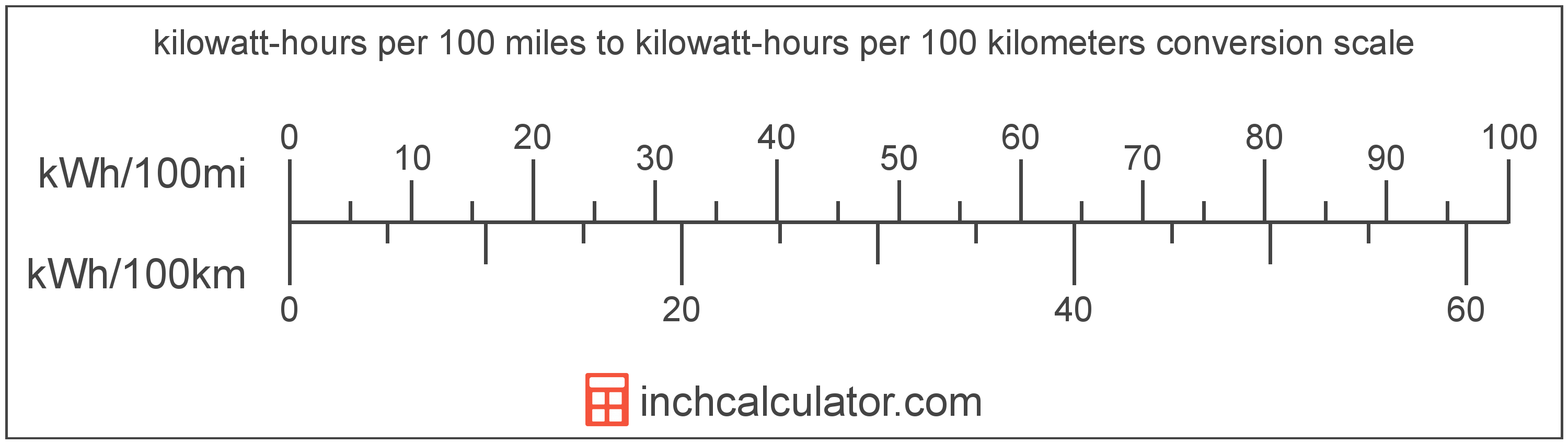# Kilowatt-Hours per 100 Kilometers to Kilowatt-Hours per 100 Miles Converter (KWh/100km to KWh/100mi)

Enter the electric car efficiency in kilowatt-hours per 100 kilometers below to get the value converted to kilowatt-hours per 100 miles.

Results in Kilowatt-Hours per 100 Miles:1 kWh/100km = 1.609344 kWh/100mi

Do you want to convert kWh/100mi to kWh/100km?

## How to Convert Kilowatt-Hours per 100 Kilometers to Kilowatt-Hours per 100 Miles

Convert kilowatt-hours per 100 kilometers to kilowatt-hours per 100 miles with this simple formula:

kWh/100mi = kWh/100km × 1.609344

Insert the kWh/100km efficiency measurement in the formula and then solve to find the result.

For example, let's convert 50 kWh/100km to kWh/100mi:

50 kWh/100km = ( 50 × 1.609344 ) = 80.4672 kWh/100miKilowatt-hours per 100 kilometers and kilowatt-hours per 100 miles are both units used to measure electric car efficiency. Keep reading to learn more about each unit of measure.

## What Are Kilowatt-Hours per 100 Kilometers?

Kilowatt-hours per 100 kilometers are a measure of electric vehicle energy efficiency equal to the kilowatt-hours of energy needed for a vehicle to travel 100 kilometers.

The kilowatt-hour per 100 kilometers is an SI unit of electric car efficiency in the metric system. Kilowatt-hours per 100 kilometers can be abbreviated as kWh/100km; for example, 1 kilowatt-hour per 100 kilometers can be written as 1 kWh/100km.

In the expressions of units, the slash, or solidus (/), is used to express a change in one or more units relative to a change in one or more other units.

## What Are Kilowatt-Hours per 100 Miles?

Kilowatt-hours per 100 miles are a measure of electric vehicle efficiency measuring the kilowatt-hours of energy required to travel 100 miles.

The kilowatt-hour per 100 miles is a US customary unit of electric car efficiency. Kilowatt-hours per 100 miles can be abbreviated as kWh/100mi; for example, 1 kilowatt-hour per 100 miles can be written as 1 kWh/100mi.

## Kilowatt-Hour per 100 Kilometers to Kilowatt-Hour per 100 Miles Conversion Table

Table showing various kilowatt-hour per 100 kilometers measurements converted to kilowatt-hours per 100 miles.
Kilowatt-hours Per 100 Kilometers Kilowatt-hours Per 100 Miles
1 kWh/100km 1.6093 kWh/100mi
2 kWh/100km 3.2187 kWh/100mi
3 kWh/100km 4.828 kWh/100mi
4 kWh/100km 6.4374 kWh/100mi
5 kWh/100km 8.0467 kWh/100mi
6 kWh/100km 9.6561 kWh/100mi
7 kWh/100km 11.27 kWh/100mi
8 kWh/100km 12.87 kWh/100mi
9 kWh/100km 14.48 kWh/100mi
10 kWh/100km 16.09 kWh/100mi
11 kWh/100km 17.7 kWh/100mi
12 kWh/100km 19.31 kWh/100mi
13 kWh/100km 20.92 kWh/100mi
14 kWh/100km 22.53 kWh/100mi
15 kWh/100km 24.14 kWh/100mi
16 kWh/100km 25.75 kWh/100mi
17 kWh/100km 27.36 kWh/100mi
18 kWh/100km 28.97 kWh/100mi
19 kWh/100km 30.58 kWh/100mi
20 kWh/100km 32.19 kWh/100mi
21 kWh/100km 33.8 kWh/100mi
22 kWh/100km 35.41 kWh/100mi
23 kWh/100km 37.01 kWh/100mi
24 kWh/100km 38.62 kWh/100mi
25 kWh/100km 40.23 kWh/100mi
26 kWh/100km 41.84 kWh/100mi
27 kWh/100km 43.45 kWh/100mi
28 kWh/100km 45.06 kWh/100mi
29 kWh/100km 46.67 kWh/100mi
30 kWh/100km 48.28 kWh/100mi
31 kWh/100km 49.89 kWh/100mi
32 kWh/100km 51.5 kWh/100mi
33 kWh/100km 53.11 kWh/100mi
34 kWh/100km 54.72 kWh/100mi
35 kWh/100km 56.33 kWh/100mi
36 kWh/100km 57.94 kWh/100mi
37 kWh/100km 59.55 kWh/100mi
38 kWh/100km 61.16 kWh/100mi
39 kWh/100km 62.76 kWh/100mi
40 kWh/100km 64.37 kWh/100mi